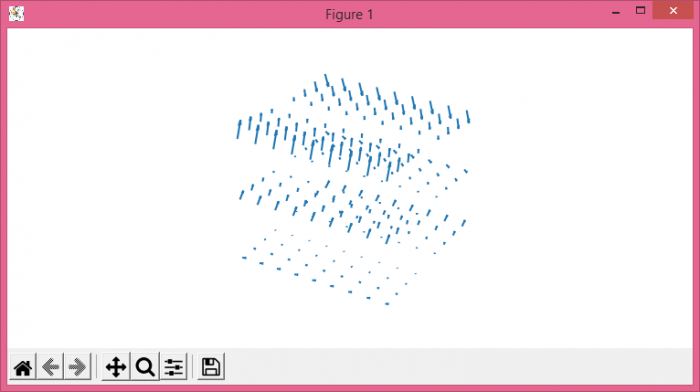# Calculate the curl of a vector field in Python and plot it with Matplotlib

MatplotlibPythonData Visualization

To calculate the curl of a vector field in Python and plot in with Matplotlib, we can use quiver() method and calculate the corresponding data.

## Steps

• Set the figure size and adjust the padding between and around the subplots.
• Create a new figure or activate an existing figure using figure() method.
• Add a 3D axes to the figure as part of a subplot arrangement.
• Create x, y and z data points using numpy meshgrid.
• Create u, v and w data curl vector positions.
• Use quiver() method to get vectors.
• Turn off the axes.
• To display the figure, use show() method.

## Example

import matplotlib.pyplot as plt
import numpy as np

plt.rcParams["figure.figsize"] = [7.50, 3.50]
plt.rcParams["figure.autolayout"] = True

fig = plt.figure()

x, y, z = np.meshgrid(np.arange(-0.8, 1, 0.2),
np.arange(-0.8, 1, 0.2),
np.arange(-0.8, 1, 0.8))

u = 0
v = y**2
w = -2*y*z - y

ax.quiver(x, y, z, u, v, w, length=0.1)
ax.axis('off')

plt.show()

## Output